English

# Are you sure you understand these tolerances?

11-02-2020

In production, if we misunderstand the tolerances marked on the drawings, it will deviate the processing analysis, processing results and requirements, and even bring serious consequences.

01 Straightness

Straightness, which is commonly referred to as flatness, indicates that the actual shape of the linear elements on the part remains in an ideal straight line. The straightness tolerance is the maximum allowable variation of the actual line to the ideal straight line.

Example 1: In a given plane, the tolerance zone must be in the area between two parallel straight lines with a distance of 0.1 mm.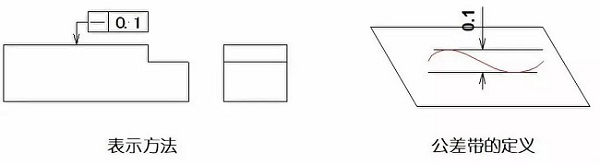Example 2: Put a mark Φ before the tolerance value, then the tolerance zone must be in the area of a cylindrical surface with a diameter of 0.08mm.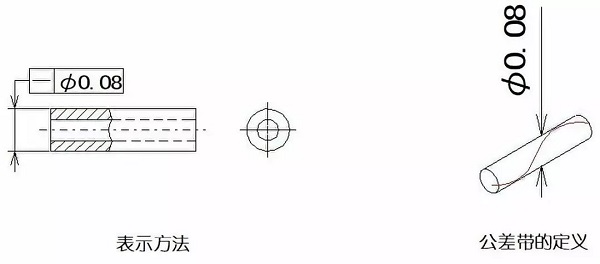02 Flatness

Flatness, the so-called flatness, indicates the actual shape of the planar elements of the part and maintains the ideal plane. The flatness tolerance is the maximum allowable variation of the actual surface from the ideal plane.

Example: The tolerance zone is the area between two parallel planes at a distance of 0.08mm.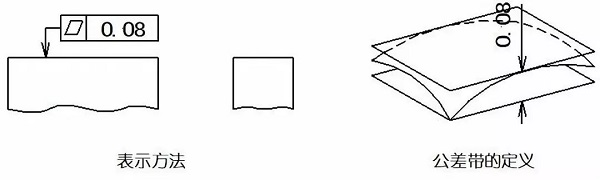03 Roundness

Roundness, which is generally called the degree of roundness, indicates that the actual shape of the elements on the part is kept at an equal distance from its center. The roundness tolerance is the maximum allowable variation between the actual circle and the ideal circle on the same section.

Example: The tolerance zone must be on the same normal section and the area between two concentric circles with a radius difference of 0.03mm.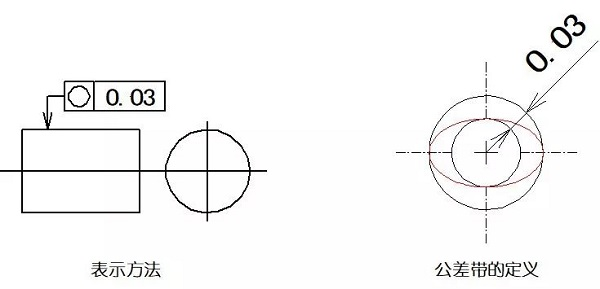04 Cylindricity

Cylindricity means that the points on the outline of the cylindrical surface on the part are kept equidistant from their axes. The cylindricity tolerance is the maximum allowable variation of the actual cylinder facing the ideal cylinder.

Example: The tolerance zone is the area between two coaxial cylindrical faces with a radius difference of 0.1mm.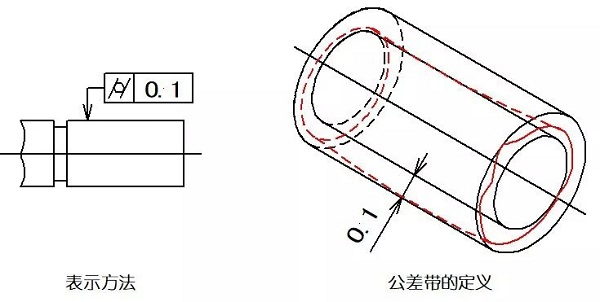05 Line Contour

Line contour is a curve of any shape on a given plane of a part to maintain its ideal shape. Line contour tolerance refers to the allowable variation of the actual contour of a non-circular curve.

Example: The tolerance zone is the area between two envelopes that envelops a series of circles with a diameter of 0.04mm tolerance. The centers of the circles lie on a line with a theoretically correct geometry.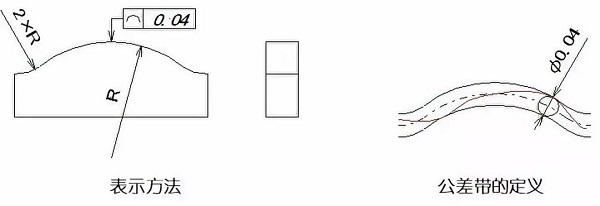06 Face Contour

Face contour is a condition that indicates a curved surface of any shape on a part and maintains its ideal shape. The surface contour tolerance refers to the actual contour of a non-circular curved surface, and the allowable variation of the ideal contour surface.

Example: The tolerance zone is formed by enveloping two envelope lines of a series of spheres with a diameter of 0.02mm. The centers of the spheres should theoretically be on the surface of the theoretically correct geometry.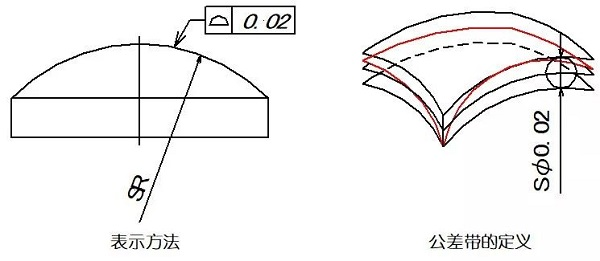07 Parallelism

Parallelism, commonly known as the degree of parallelism, indicates that the actual elements measured on the part are kept at equal distances from the reference. The parallelism tolerance is the maximum allowable variation between the actual direction of the measured element and the ideal direction parallel to the reference.

Example: If the mark Φ is added before the tolerance value, the tolerance zone is within the cylindrical surface of the reference parallel diameter Φ0.03mm.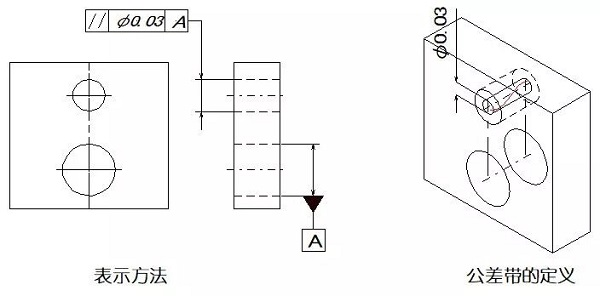08 verticality

The perpendicularity, which is generally referred to as the degree to which the two elements remain orthogonal, indicates that the measured element on the part maintains a correct 90 ° angle relative to the reference element. The perpendicularity tolerance is the maximum allowable variation between the actual direction of the measured element and the ideal direction perpendicular to the reference.

Example 1: Put a mark Φ in front of the tolerance zone, then the tolerance zone is perpendicular to the cylindrical surface with a diameter of 0.1mm on the reference plane.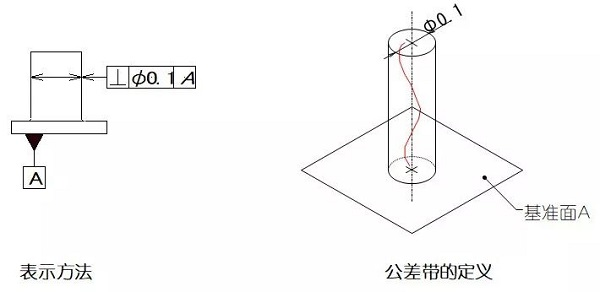Example 2: The tolerance zone must be between two parallel planes with a distance of 0.08 mm and perpendicular to the reference line.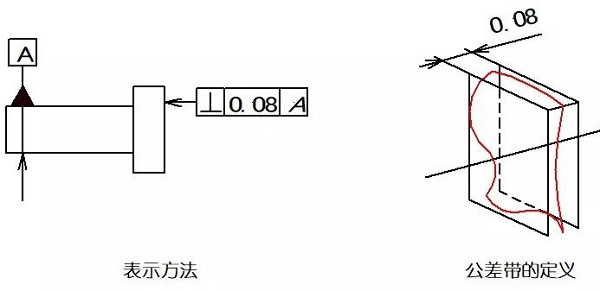09 Tilt

The inclination is the correct condition to indicate that the two elements on the part are facing in any given angle relative to each other. The inclination tolerance is the maximum allowable amount of variation between the actual orientation of the measured element and the ideal orientation of the reference at any given angle.

Example 1: The tolerance zone of the measured axis is the area between two parallel planes with a distance of 0.08 mm and a theoretical angle of 60 ° from the reference plane A.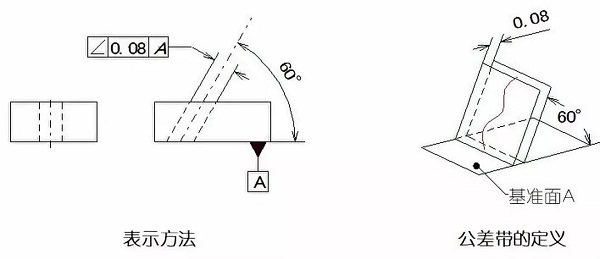Example 2: Put a mark Φ before the tolerance value, then the tolerance zone must be within a cylindrical surface with a diameter of 0.1mm. The tolerance zone should be parallel to plane B perpendicular to datum A and at a theoretically correct angle of 60 ° with datum A.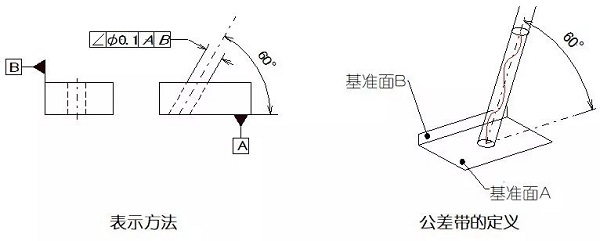10 Position degree

The degree of position is the accuracy of the points, lines, and areas on the part relative to their ideal position. Position tolerance is the maximum allowable variation of the actual position of the measured element relative to the ideal position.

Example: When the mark SΦ is added before the tolerance zone, the tolerance zone is the area inside the ball with a diameter of 0.3mm. The position of the center point of the ball tolerance zone is relative to the theoretically correct dimensions of the reference A, B, and C.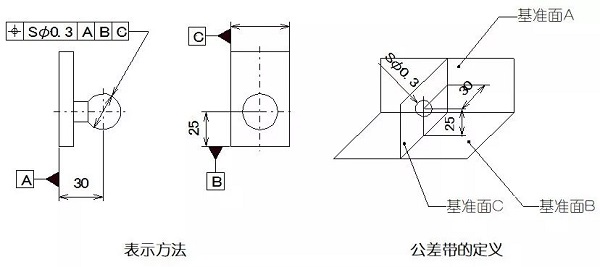11 Coaxial (concentric) degree

Concentricity, commonly referred to as the degree of coaxiality, indicates that the measured axis of the part is kept on the same straight line relative to the reference axis. The coaxiality tolerance is the allowable variation of the measured actual axis with respect to the reference axis.

Example: When the tolerance value is marked, the tolerance zone is the area between cylinders with a diameter of 0.08mm. The axis of the circular tolerance zone corresponds to the datum.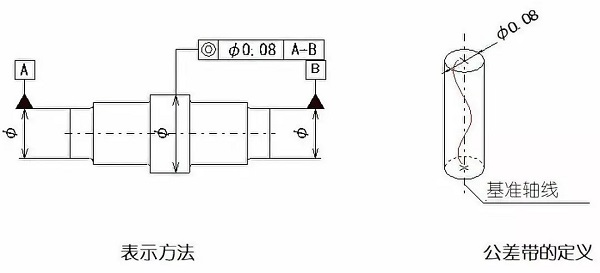12 Symmetry

Symmetry is a state in which two symmetrical center elements on a part are kept in the same center plane. The symmetry tolerance is the allowable variation of the symmetry center plane (or center line, axis) of the actual element to the ideal symmetry plane.

Example: The tolerance zone is the area between two parallel planes or straight lines with a distance of 0.08 mm and symmetrically arranged with respect to the reference center plane or center line.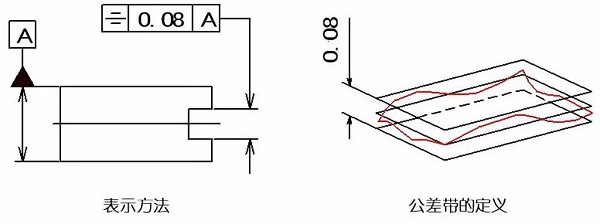13 Round beating

Circular runout is a condition where the rotating surface on the part is within a limited measuring plane and remains in a fixed position relative to the reference axis. The round runout tolerance is the maximum allowable variation within the limited measurement range when the measured actual element rotates a full circle without axial movement around the reference axis.

Example 1: The tolerance zone is the area between two concentric circles with a radius difference of 0.1 mm and the center of the circle on the same reference axis perpendicular to any measurement plane.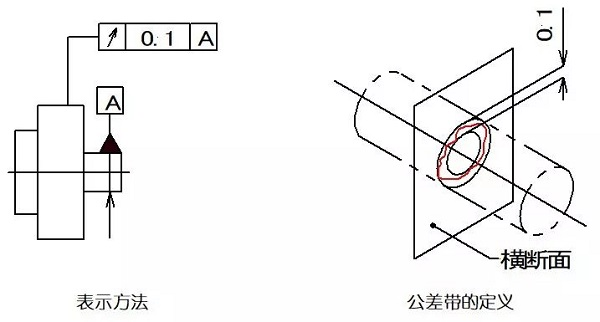Example 2: The tolerance zone is the area between two circles with a distance of 0.1 mm on the measuring cylindrical surface at any radial position coaxial with the reference.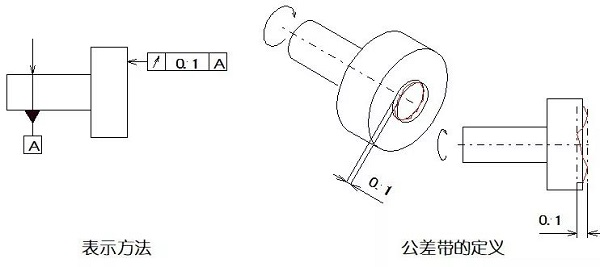14 Full beating

Full runout refers to the amount of runout along the entire measured surface when the part is continuously rotated around the reference axis. Full runout tolerance is the maximum allowable runout of the measured actual element when it is continuously rotated around the reference axis while the indicator is relatively moving along its ideal contour.

Example 1: The tolerance zone is the area between two cylindrical surfaces that have a radius difference of 0.1 mm and are coaxial with the reference.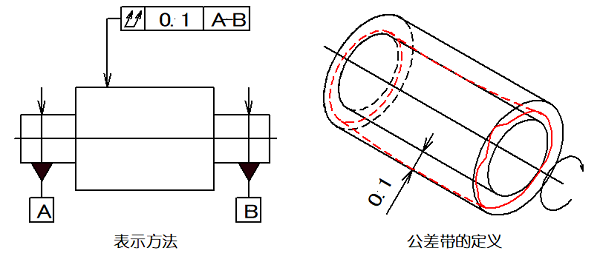Example 2: The tolerance zone is the area between two parallel planes with a distance of 0.1 mm in radius and perpendicular to the datum.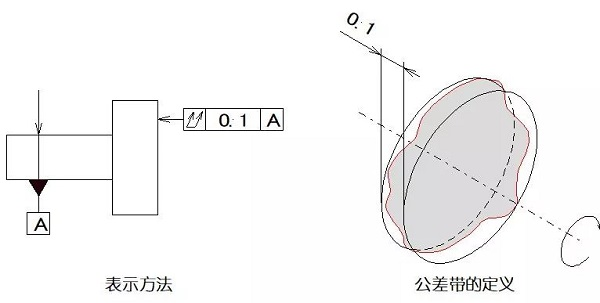Get the latest price? We'll respond as soon as possible(within 12 hours)